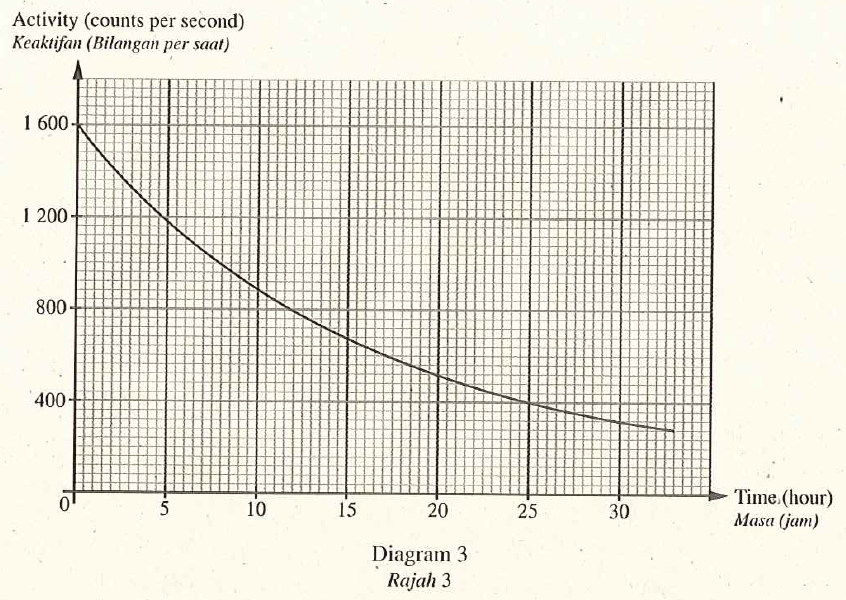# Structured Questions – Half Life

Diagram 3 shows the activity-time graph of radioisotope Potassium-42. This radioisotope emits beta particles and is used as a tracer to measure the quantity of salt in human body.1. State one characteristic of beta particles. [1 mark]
Negatively charged
2. Based on Diagram 3 , determine the half-life of Potassium-42. Show on the graph how you determine the half-life of Potassium. [2 mark]
12 hours
3. Based on the half life, state why Potassium-42 is suitable to be used as the tracer. [1 mark]
Potassium-42 has short half-life, hence overexposure does not occur.
4. Calculate the time taken for radioistope Potassium-42 to reduce $$\frac{1}{8}$$ of its initial activity. [2 marks]
$$1 \underset{ T _{\frac{1}{2}}}{\longrightarrow} \frac{1}{2} \underset{ T _{\frac{1}{2}}}{\longrightarrow} \frac{1}{4} \underset{ T _{\frac{1}{2}}}{\longrightarrow} \frac{1}{8}$$
The time taken for the activity of the potassium- 42 becomes $$\frac{1}{8}$$ of its initial activity.
\begin{aligned} &=3 T _{\frac{1}{2}} \\ &=3 \times 12 \text { hours } \\ &=36 \text { hours } \end{aligned}